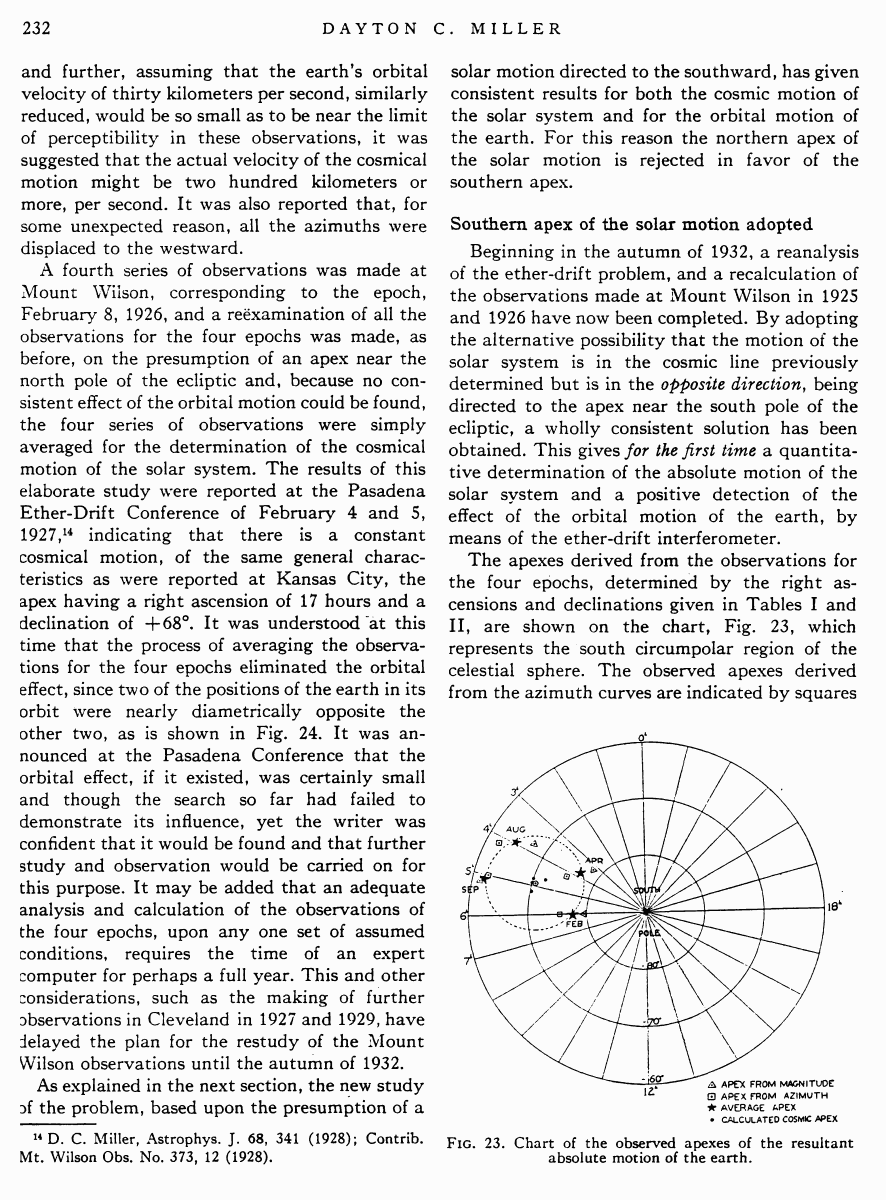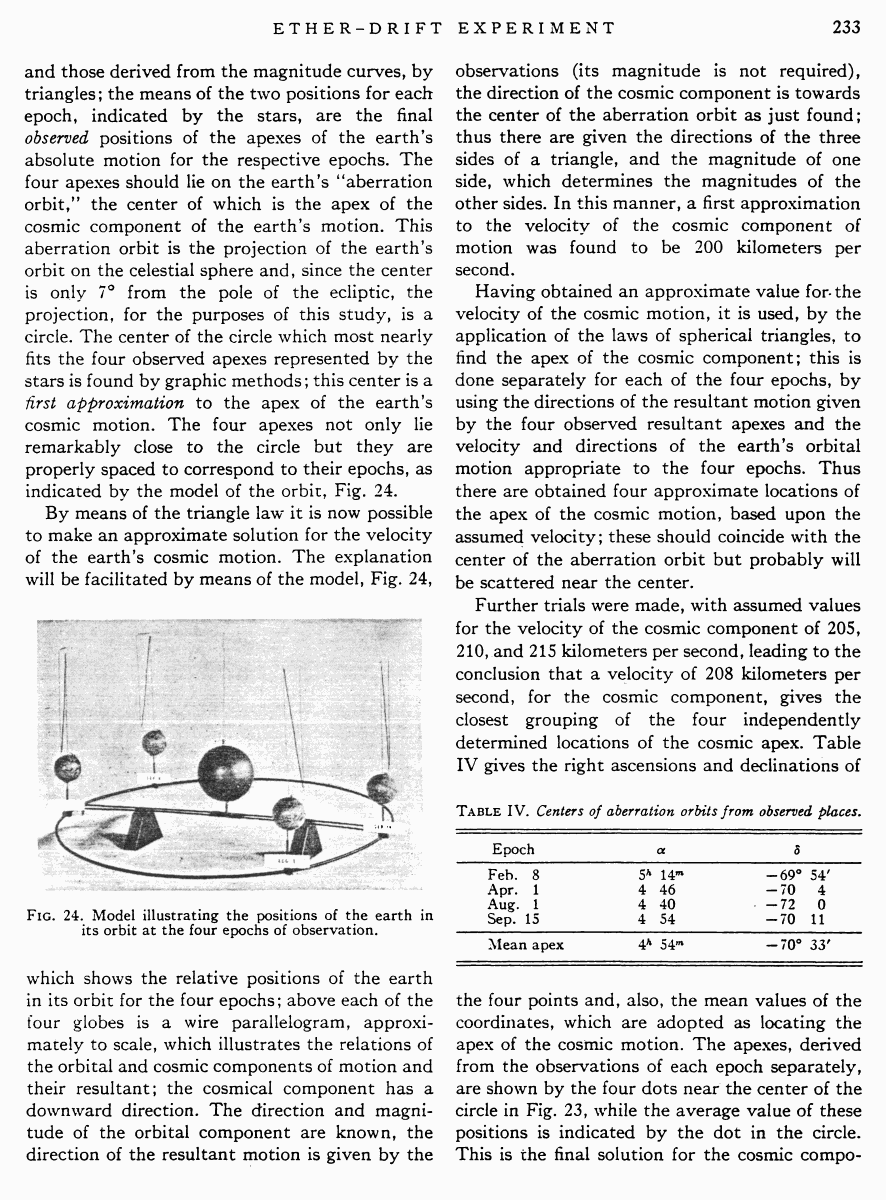# Miller D.C. The Ether-Drift Experiment and the Determination of the Absolute Motion of the Earth // Reviews of modern physics, Vol.5, July 1933

Start   PDF   <<<  Page 232   >>>203  204  205  206  207  208  209  210  211  212  213  214  215  216  217  218  219  220  221  222  223  224  225  226  227  228  229  230  231  232 233  234  235  236  237  238  239  240  241  242

and further, assuming that the earthÆs orbital velocity of thirty kilometers per second, similarly reduced, would be so small as to be near the limit of perceptibility in these observations, it was suggested that the actual velocity of the cosmical motion might be two hundred kilometers or more, per second. It was also reported that, for some unexpected reason, all the azimuths were displaced to the westward.

A fourth series of observations was made at Mount Wilson, corresponding to the epoch, February 8, 1926, and a reexamination of all the observations for the four epochs was made, as before, on the presumption of an apex near the north pole of the ecliptic and, because no consistent effect of the orbital motion could be found, the four series of observations were simply averaged for the determination of the cosmical motion of the solar system. The results of this elaborate study were reported at the Pasadena Ether-Drift Conference of February 4 and 5,

1927,14 indicating that there is a constant cosmical motion, of the same general characteristics as were reported at Kansas City, the apex having a right ascension of 17 hours and a declination of +68░. It was understood at this time that the process of averaging the observations for the four epochs eliminated the orbital effect, since two of the positions of the earth in its orbit were nearly diametrically opposite the other two, as is shown in Fig. 24. It was announced at the Pasadena Conference that the orbital effect, if it existed, was certainly small and though the search so far had failed to demonstrate its influence, yet the writer was confident that it would be found and that further study and observation would be carried on for this purpose. It may be added that an adequate analysis and calculation of the observations of the four epochs, upon any one set of assumed conditions, requires the time of an expert computer for perhaps a full year. This and other considerations, such as the making of further observations in Cleveland in 1927 and 1929, have delayed the plan for the restudy of the Mount Wilson observations until the autumn of 1932.

As explained in the next section, the new study of the problem, based upon the presumption of a

14 D. C. Miller, Astrophys. J. 68, 341 (1928); Contrib. Mt. Wilson Obs. No. 373, 12 (1928).

solar motion directed to the southward, has given consistent results for both the cosmic motion of the solar system and for the orbital motion of the earth. For this reason the northern apex of the solar motion is rejected in favor of the southern apex.

Southern apex of the solar motion adopted

Beginning in the autumn of 1932, a reanalysis of the ether-drift problem, and a recalculation of the observations made at Mount Wilson in 1925 and 1926 have now been completed. By adopting the alternative possibility that the motion of the solar system is in the cosmic line previously determined but is in the opposite direction, being directed to the apex near the south pole of the ecliptic, a wholly consistent solution has been obtained. This gives for the first time a quantitative determination of the absolute motion of the solar system and a positive detection of the effect of the orbital motion of the earth, by means of the ether-drift interferometer.

The apexes derived from the observations for the four epochs, determined by the right ascensions and declinations given in Tables I and II, are shown on the chart, Fig. 23, which represents the south circumpolar region of the celestial sphere. The observed apexes derived from the azimuth curves are indicated by squares

Fig. 23. Chart of the observed apexes of the resultant absolute motion of the earth.

and those derived from the magnitude curves, by triangles; the means of the two positions for each epoch, indicated by the stars, are the final observed positions of the apexes of the earthÆs absolute motion for the respective epochs. The four apexes should lie on the earthÆs ōaberration orbit,ö the center of which is the apex of the cosmic component of the earthÆs motion. This aberration orbit is the projection of the earthÆs orbit on the celestial sphere and, since the center is only 7░ from the pole of the ecliptic, the projection, for the purposes of this study, is a circle. The center of the circle which most nearly fits the four observed apexes represented by the stars is found by graphic methods; this center is a first approximation to the apex of the earthÆs cosmic motion. The four apexes not only lie remarkably close to the circle but they are properly spaced to correspond to their epochs, as indicated by the model of the orbit, Fig. 24.

By means of the triangle law it is now possible to make an approximate solution for the velocity of the earthÆs cosmic motion. The explanation will be facilitated by means of the model, Fig. 24,

Fig. 24. Model illustrating the positions of the earth in its orbit at the four epochs of observation.

which shows the relative positions of the earth in its orbit for the four epochs; above each of the four globes is a wire parallelogram, approximately to scale, which illustrates the relations of the orbital and cosmic components of motion and their resultant; the cosmical component has a downward direction. The direction and magnitude of the orbital component are known, the direction of the resultant motion is given by the

observations (its magnitude is not required), the direction of the cosmic component is towards the center of the aberration orbit as just found; thus there are given the directions of the three sides of a triangle, and the magnitude of one side, which determines the magnitudes of the other sides. In this manner, a first approximation to the velocity of the cosmic component of motion was found to be 200 kilometers per second.

Having obtained an approximate value for the velocity of the cosmic motion, it is used, by the application of the laws of spherical triangles, to find the apex of the cosmic component; this is done separately for each of the four epochs, by using the directions of the resultant motion given by the four observed resultant apexes and the velocity and directions of the earthÆs orbital motion appropriate to the four epochs. Thus there are obtained four approximate locations of the apex of the cosmic motion, based upon the assumed velocity; these should coincide with the center of the aberration orbit but probably will be scattered near the center.

Further trials were made, with assumed values for the velocity of the cosmic component of 205, 210, and 215 kilometers per second, leading to the conclusion that a velocity of 208 kilometers per second, for the cosmic component, gives the closest grouping of the four independently determined locations of the cosmic apex. Table IV gives the right ascensions and declinations of

Table IV. Centers of aberration orbits from observed places.

 Epoch α δ Feb. 8 5h 14m ¢69░ 54′ Apr. 1 4 46 ¢70 4 Aug. 1 4 40 ¢72 0 Sep. 15 4 54 ¢70 11 Mean apex 4h 54m ¢70░ 33′

the four points and, also, the mean values of the coordinates, which are adopted as locating the apex of the cosmic motion. The apexes, derived from the observations of each epoch separately, are shown by the four dots near the center of the circle in Fig. 23, while the average value of these positions is indicated by the dot in the circle. This is the final solution for the cosmic compo